Transcripted Summary

In this chapter we'll cover some fundamentals.

We'll take our algorithm and turn it into code, which is another word for programs. And we'll learn about packages, classes, methods, data types, variables, input and output, naming conventions, and comments.

Let's go!

Here I've opened IntelliJ, which is our editor — this is what we'll write our programs in.

Don’t have IntelliJ installed yet?

There's a link in the resources that will bring you to where you need to go in order to download and install IntelliJ.

Once you have that installed, open it up and then choose to Create a New Project.

Now, you'll be able to select the type.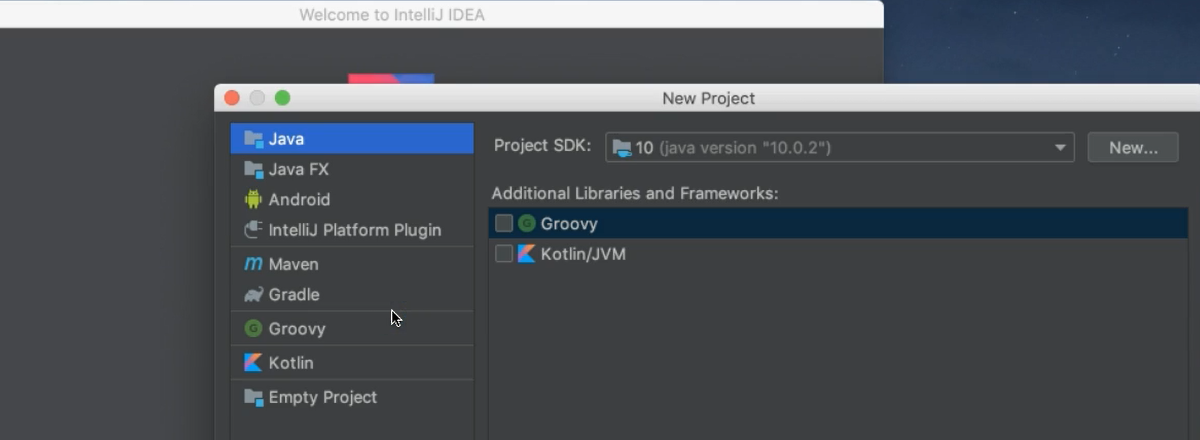• The type we're going to select is a Java project, and then click Next
• Make sure that you don't have a template selected and click Next
• Then we need to give it a project name. We'll just call this “java_course”, and then click Finish

Now on the left, you'll see the project view — this is where all of our files live.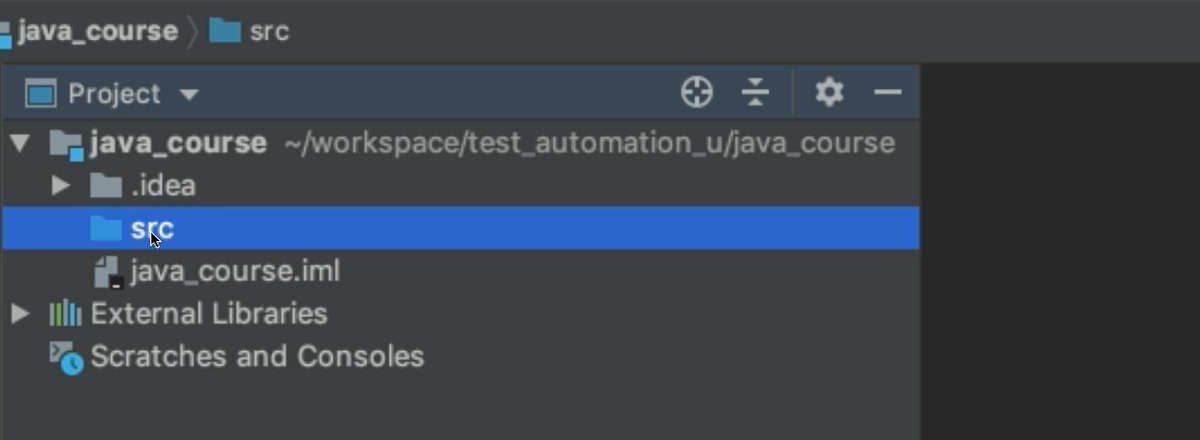So far, we don't have much because this is a new project.

But we can add things to this source (src) folder. Source code is also a term that's used for programs.

• So, under this src folder, we're going to right-click
• We're going to click New, and we're going to choose to add a Package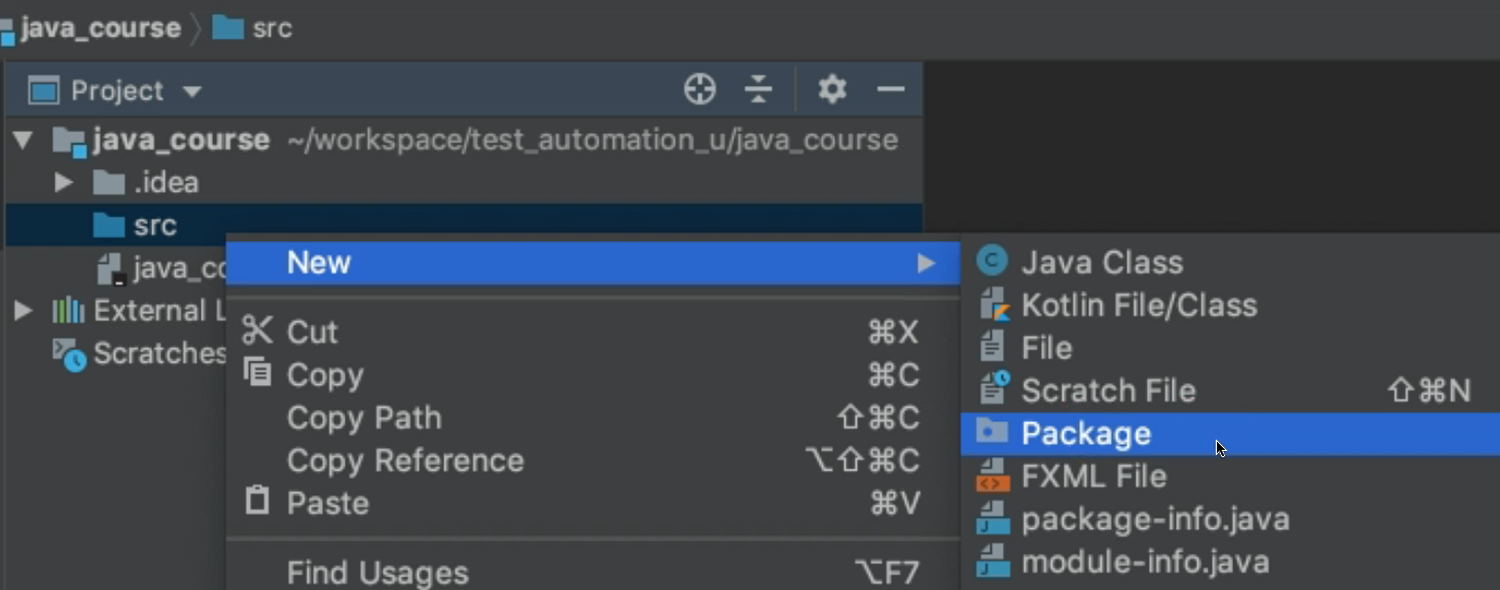Packages

A package in Java is a folder/container that holds Java files.

You can have multiple packages. In fact, you should, depending on the relationship between the code. So, make logical decisions about your packages.

So, we're going to create our first package. And we need to give it a name.

Now for Java packages, the convention is that the packages should be all lowercase. This is not a rule - meaning your programs won’t break if you don’t follow this. However, this is a convention.

Java Programming Conventions

When I say convention throughout the course, this means this is what's been adopted and accepted by Java programmers worldwide. Following these conventions makes it easy for you to know what's what within your program, as well as other programmers who have to read or edit your code.

Let's go ahead and give it a package name.

We're going to call this `chapter2`.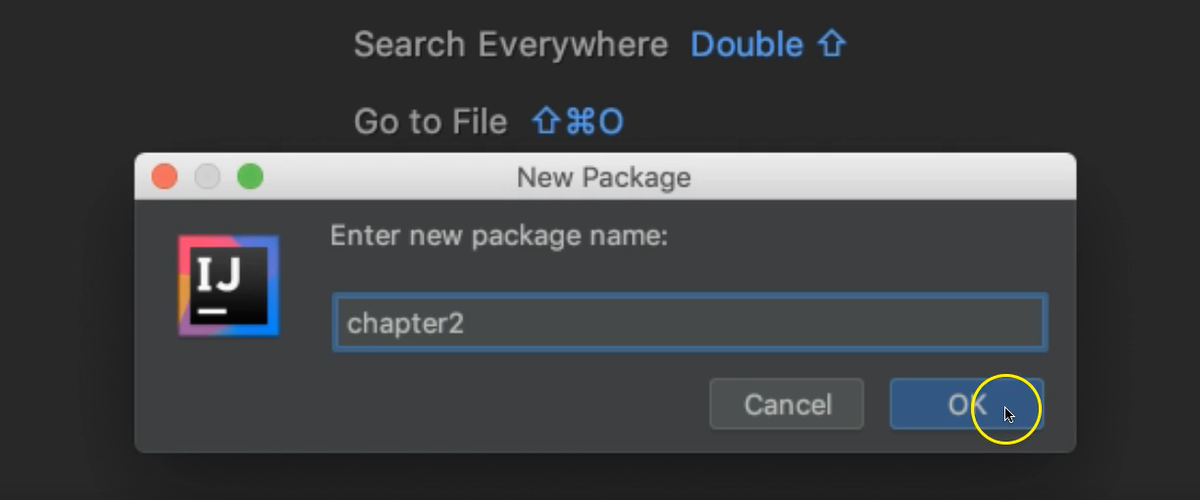And that's a logical name for a folder that will hold all of our files that we create in Chapter 2.

We'll click OK, and then if we expand this src folder we see our new package, which is `chapter2`. Notice there's a little circle on here to denote a package.

Now, packages again contain Java files, also known as classes.

So, we're going to right-click on here (chapter2), we're going to select New, and then choose Java Class

We're going to make a class that will implement the algorithm we looked at in the last chapter which calculates the gross pay.

So, we're going to name our class something that is reflective of the tasks that it needs to do.

So, we'll call this: `GrossPayCalculator`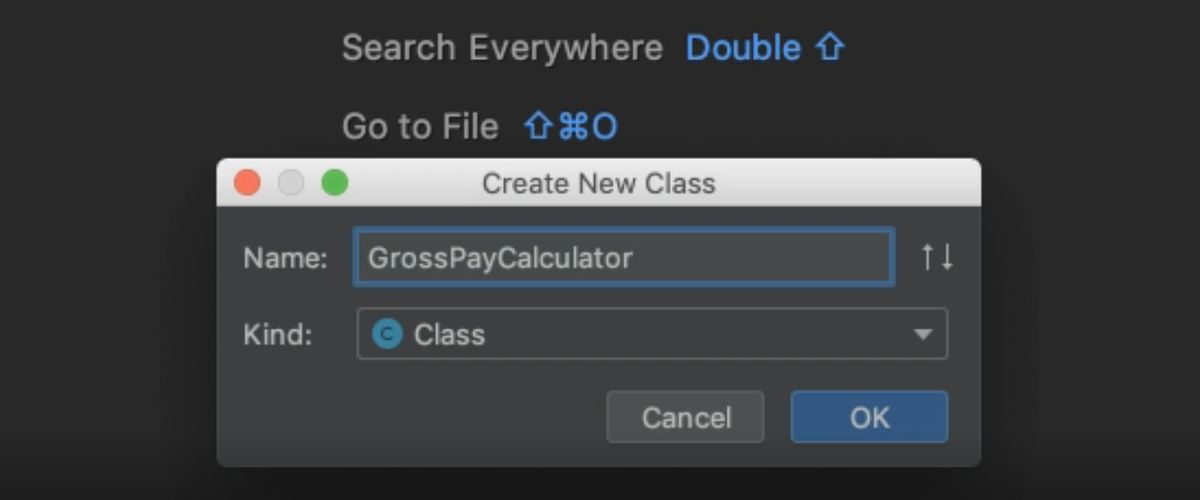Notice here, I'm using three words to describe this class. However, there are no spaces.

That's because Java does not allow spaces in the name of a class. Also, notice that within here, although it's three different words and they're all together, each word begins with a capital letter. That's for readability, and it's also a convention within Java.

So, we click OK, and we notice now we have a new class. This is our Java file.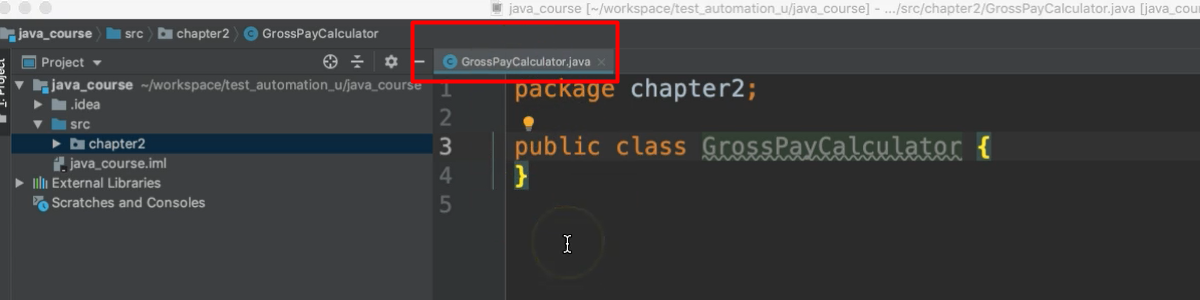Notice the name in this tab here, “GrossPayCalculator.java”. All Java files end with a `.java` extension.

Now I'm going to double-click this tab just so that it makes it bigger in our editor. Notice that the very first line of the Java file is the package declaration.

If your Java file is within a package, which ours is, then the first line must declare what package that is, followed by a semicolon.

So, we simply write `package` and then give it the name that we already named our package (chapter2).

``````package chapter2;
``````

And then the next line must be the class declaration. So, within a Java file, you have a Java class.

The Java class is declared as: `public class GrossPayCalculator`

And there's 2 curly braces. In Java, the curly braces are used to encompass a block of code.

``````package chapter2;

public class GrossPayCalculator {

}
``````

Notice that some of these words are in orange (in IntelliJ) — `package`, `public`, `class` — these are in orange because these are reserved words in Java. And our editor has chosen this color for reserved words.

Reserved Words in Java

Reserved words are words that this programming language has decided means something specific within the language and therefore cannot be used to name things within our program. So, we couldn't name something “class”, or “public” or “package” because these words are reserved.

Now let's go inside these curly braces.

Everything that we put between these 2 braces will belong to this Java class. This is a block of code. Inside of here, we're going to create a method.

Now in order to run any code from within a class, it must be inside of a method.

So, we're going to create the entry-point method in Java:

``````package chapter2;

public class GrossPayCalculator {

public static void main(String[] args){

}
}
``````

This `main` method, like I said, it is the entry point for running a program in Java.

When we execute this class, what it's going to do is first look for the `main` method within this class, and this is the starting point of the program. And it has to be defined just like this.

It also has a set of curly braces. So, everything that exists between these curly braces will belong to this `main` method.

And we'll talk more about what all of this stuff means in later chapters. But for now, in this chapter I just want us to focus on implementing that algorithm.

I've copied the steps of our algorithm, and I'm just going to paste them inside of our method.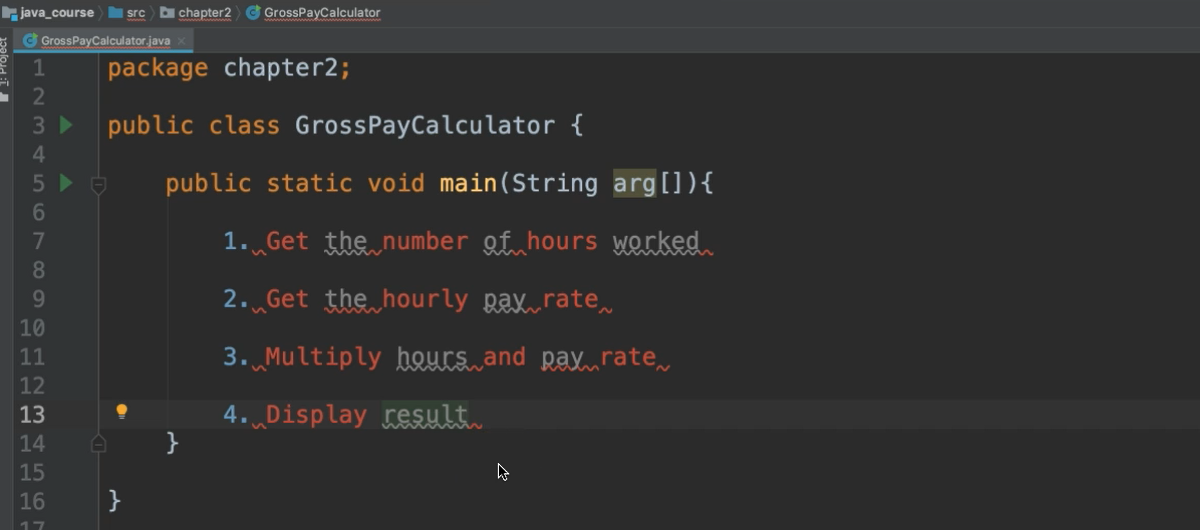Now notice, when I paste them here, there is some red text and some squiggly lines.

These are errors, also known as compilation errors. What this means is Java has no clue of what I'm talking about, because this is not Java, this is English.

So, I'm going to go ahead and comment these lines out.

To comment out a line, you simply add 2 slashes (//), and that will turn that line into a comment:

``````//1. Get the number of hours worked
``````

What is a Java Comment?

A comment is something for the programmer, for humans to read, not the computers. So, the computer will ignore lines that are commented out, and will not try to process them. So, these are just for the programmers.

Okay, so let's do that for each one of these.

``````package chapter2;

public class GrossPayCalculator {

public static void main(String[] args){

//1. Get the number of hours worked

//2. Get the hourly pay rate

//3. Multiply hours and pay rate

//4. Display result
}
}
``````

This will just serve as guidance to help us as we're programming this algorithm.

So, the very first thing that we want to do is to get the number of hours worked. Where do we get this from? Well, we can simply ask the user for this information.

The way we're going to ask them is to use output.

And in Java to display output to the console, we use:

``````System.out.println()
``````

Inside of these parentheses, we add what's called a String. A String represents text, and is enclosed within quotation marks (" "). So, what do we want the user to see?

We want to ask them how many hours did the employee work.

``````System.out.println("Enter the number of hours the employee worked.");
``````

A statement is essentially one instruction. So, this is one statement that's saying to print this String to the console. And then we have this semicolon which is used to end a statement in Java. Every statement ends with a semicolon.

``````package chapter2;

public class GrossPayCalculator {

public static void main(String[] args){

//1. Get the number of hours worked
System.out.println("Enter the number of hours the employee worked.");

//2. Get the hourly pay rate

//3. Multiply hours and pay rate

//4. Display result
}
}
``````

We've asked them to enter the hours worked; now we need to receive that information as input so that we can use it in our calculation.

To receive input in Java from the console we'll use this built-in Java class, `Scanner`. To read from the input:

``````Scanner scanner = new Scanner(System.in);
``````

Now don't worry too much about this line for now, this is essentially just used to create an object to scan in information.

Now we do see the Scanner word is red, meaning we do have a compilation error.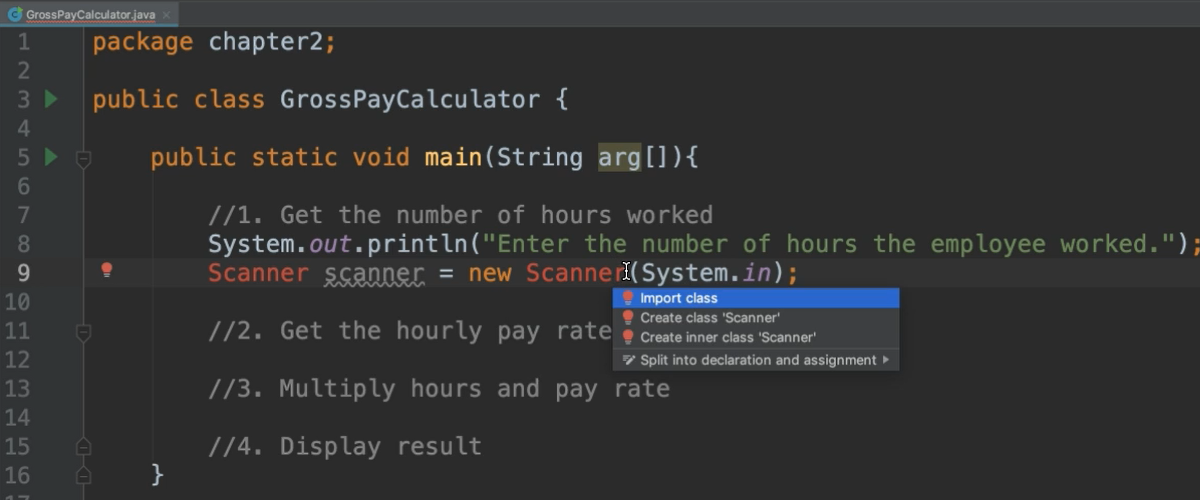This is because we need to import this Scanner class.

To import the class, you can click the red light bulb on the left and select the option to import the class. There are shortcuts to quickly add the imports as well:

1. On Mac, hold down the option and return key at the same time

2. On Windows, hold down the Alt key and the Enter key at the same time

Import statements go between the package declaration and the class declaration.

``````package chapter2;

import java.util.Scanner;

public class GrossPayCalculator {
``````

That's the first part to get the input; we still have to actually read it in.

So, we use the `Scanner` and there's a bunch of methods here that we can choose from. We want to use one of these `next` methods.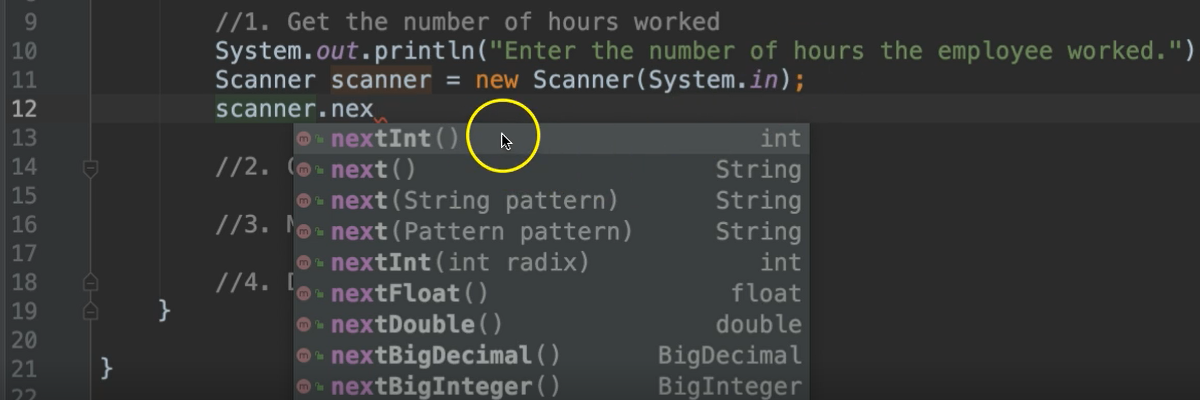The one you use depends on which type of data that you're trying to receive. We are asking the users to enter the number of hours they worked. Therefore, we know we're looking for a number.

This first one `nextInt` lets us know that this will read an integer. And you can see what type of data that it deals with. All of these are known as data types. And data types are really important. We'll talk about those in a little bit.

So, the number of hours worked. Let's assume that this is a whole number.

I'm going to use:

``````scanner.nextInt();
``````

And this will read that data in.

``````package chapter2;

import java.util.Scanner;

public class GrossPayCalculator {

public static void main(String[] args){

//1. Get the number of hours worked
System.out.println("Enter the number of hours the employee worked.");
Scanner scanner = new Scanner(System.in);
scanner.nextInt();
``````

Now let's go on to our next step. We want to get the hourly pay rate.

This will be very similar to this where we first prompt the user with output and then we receive the input. So let's say:

``````System.out.println`("Enter the employee's pay rate.");
``````

Now we want to use this `scanner` again to receive the input. We've already declared the `scanner` itself on line 11, so we don't have to worry about that again.

We'll just say `scanner.`, and this time let's see which method is most appropriate.

This one is the pay rate, which is money. So this is going to be a number, but it has a decimal in it. So we can use one of these: `nextFloat` or `nextDouble`.

`double` is most commonly used for everyday usage. So, we'll say:

``````scanner.nextDouble();
``````

Now we're ready for step 3, which is to multiply the hours by the pay rate.

So, we want to take the information that we got on line 12, along with the information that we got on line 16 and multiply those things together for a result. But we have a problem here. We haven't stored this information. We read it in, but there's no way to reference it.

That's because we didn't use a variable.

Declaring Variables

A variable is essentially a memory location. This stores data. And variables can store all sorts of data. However, you must declare what type of data that it should store, because different types of data use different amounts of memory.

So, for this integer, we know that this is an `int`, we declare it by using the `int` data type, followed by a variable name.

I'm going to call this, `hours`, and then we put an equal sign to let it know to store this information inside of this variable:

``````int hours = scanner.nextInt();
``````

So again, we declare this variable with the data type of int. We've given it a name of hours, and then we put an equals sign, followed by the data that it holds.

This is a variable declaration.

Always the data type and the name of the variable go on the left side of the equals sign. The value that you want to store inside of this memory location goes on the right side of the equals sign.

And then we want to do the same thing with line 16. This time it's a `double`, so we use the `double` data type.

We give this a name, let's call it `rate`:

``````double rate = scanner.nextDouble();
``````

Notice that I named these variables "hours" and "rate" to represent the information that they're holding. When I want to use these later on, it will be clear to me what they represent.

Importance of Descriptive Names

A lot of times people will be lazy and name their variables in abbreviated words or even letter (e.g., “h” for hours or “r” for rate). This is discouraged. Please be descriptive when you're naming your variables, methods, classes, and packages. Anything that you name in programming, be descriptive of what it represents, because whenever you look at this again, you don't want to have to guess or dig around to figure out what it represents. Also, when you're collaborating with other programmers, they may have to update your program later, so it's very helpful when things are named appropriately.

Back to the code.

We want to multiply the hours and the pay rate, and we're going to also store this in a variable. So, what should be the data type of this variable, we ask ourselves.

Well, we're multiplying an integer by a decimal number.

This could very well be a decimal number. So that's the data type that we want to use to hold this result. So, we say:

``````double grossPay = hours * rate;
``````

Multiplication in Java is the asterisk symbol.

Notice, we use the exact same names of the variables `hours` and `rate` that we declared earlier. They have to be exactly the same when used later. Java is case sensitive.

So, if I declared the variable as `hours` but then later referred to it as `Hours`, this would become red - meaning it’s a compilation error. If I hover over the error, I see it says “cannot resolve symbol Hours”. Meaning it doesn’t know what this `Hours` is, because the case doesn’t match. So you have to be careful with that.

Also notice that I named the newest variable `grossPay` with a capital "P". Just like we talked about with the class names, it's camelCase, but variables start with lowercase.

Now we want to display the result.

So, we put:

``````System.out.println(grossPay);
``````

One more thing, after we read in the input, we should close this `scanner` to let Java know we’re done with it and that they can free up that memory space.

``````scanner.close();
``````

### # GrossPayCalculator.java

``````package chapter2;

import java.util.Scanner;

public class GrossPayCalculator {

public static void main(String arg[]){

//1. Get the number of hours worked
System.out.println("Enter the number of hours the employee worked.");
Scanner scanner = new Scanner(System.in);
int hours = scanner.nextInt();

//2. Get the hourly pay rate
System.out.println("Enter the employee's pay rate.");
double rate = scanner.nextDouble();
scanner.close();

//3. Multiply hours and pay rate
double grossPay = hours * rate;

//4. Display result
System.out.println(grossPay);
}
}
``````

Now let's go ahead and run our program.

To run this program, you _right-click _anywhere within the class, and then select this option to Run.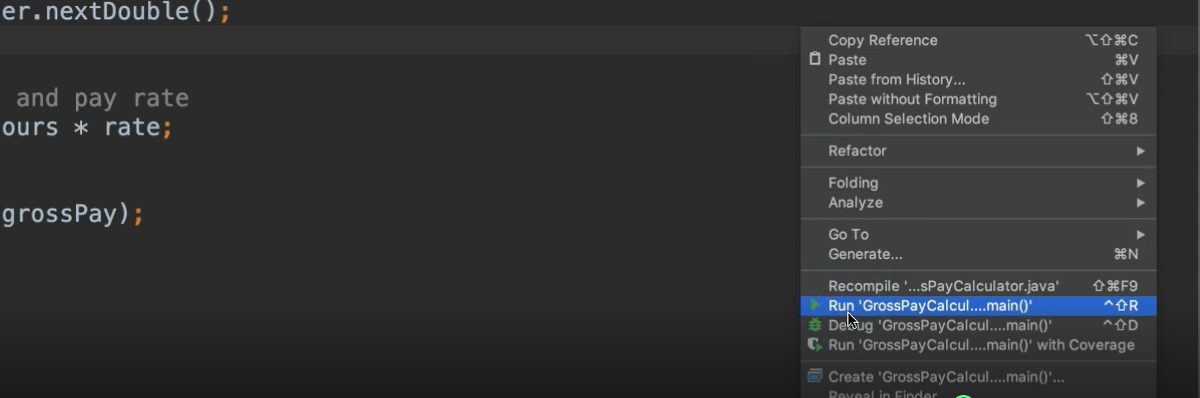Notice a console now opens and it prints out the sentence or the String that we asked it to, “Enter the number of hours worked”.

Let's respond with 40.

And then it says “Enter the employee's pay rate”.

Let’s enter 20.

Notice it prints out 800, so perfect.

Now, when you're programming, I want you to always keep in mind who you're programming for.

In this case, we are implementing something where we want someone to enter this information and then we print out. Now there's nowhere on the console that lets them know what this number represents, that this is the gross pay.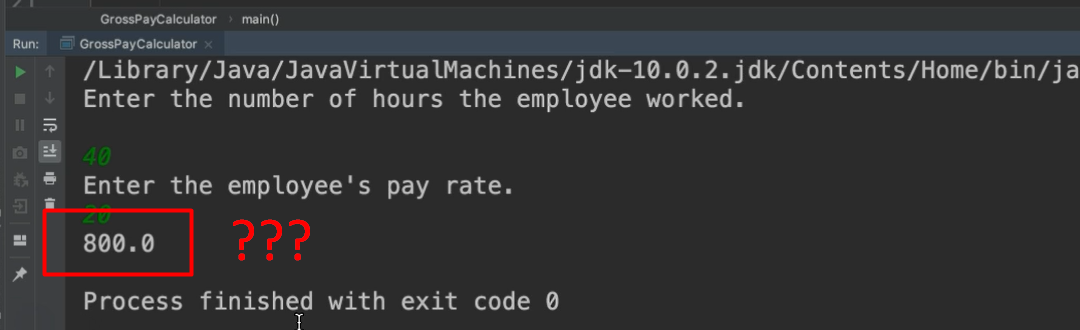Whoever is using this, they probably wouldn’t be using it from a console. It will be somewhere else like maybe on a webpage or something. So, they have no insight into what's going on in this code. So, from their standpoint all they see is the output that we see in our console. So, it’s best to be descriptive.

So, instead of only printing out the gross pay, we're going to print out some text along with it.

We'll say, “The employee’s gross pay is \$”, and then we can add a plus sign and the variable that we want to append the value of to the end of this String.

``````System.out.println("The employee's gross pay is \$" + grossPay);
``````

Now we can run this.

After entering 40 and 20 again for input, we now see a nice sentence letting us know what's going on.

``````The employee’s gross pay is \$800.0
``````

### # Before we close out this chapter, there's a little bit more I want to go over as far as variable names.

There are some things that you can do with variable names:

• You can add numbers to variable names. However, the numbers cannot be the very first character of the variable name. Notice now `1hour` turns to red.

• Also, you can add special characters like \$ or _, but there are some special characters that are not allowed like - for example. So just be careful when you're naming your variables. If you run into any issues, the compiler should let you know.

## Optional Independent Exercise

To practice what you’ve learned in this chapter, here is an exercise for you to try on your own.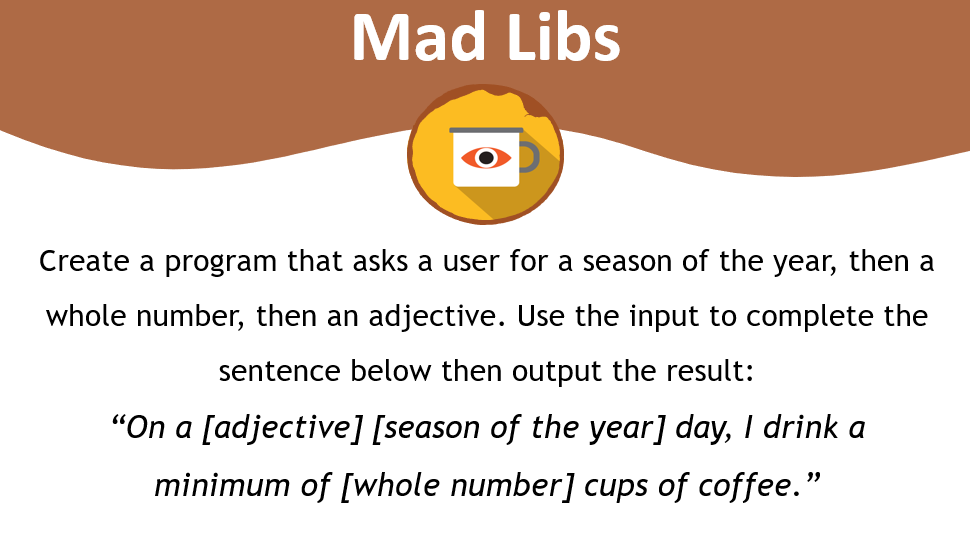Create a program that asks a user for a season of the year, then a whole number, then an adjective. And use the input to complete the sentence below. Output the result.

Good luck!

Solution

Programming can be done many different ways, but here’s my solution.